Strongly Connected Components

# Strongly Connected Components

#### In this tutorial, you will learn how strongly connected components are formed. Also, you will find working examples of kosararju's algorithm in C, C++, Java and Python.

A strongly connected component is the portion of a directed graph in which there is a path from each vertex to another vertex. It is applicable only on a directed graph.

For example:

Let us take the graph below.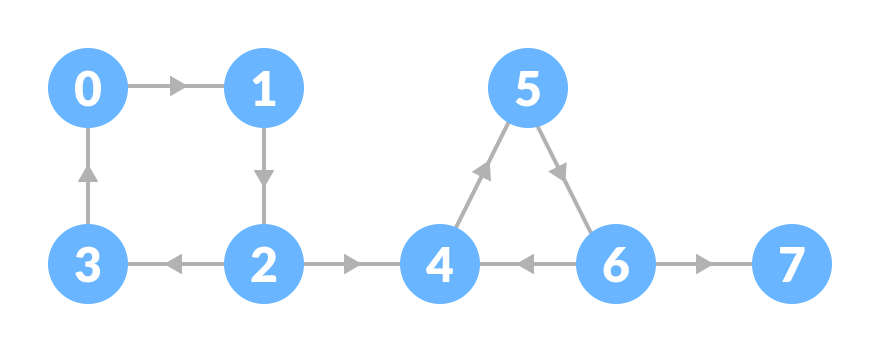The strongly connected components of the above graph are: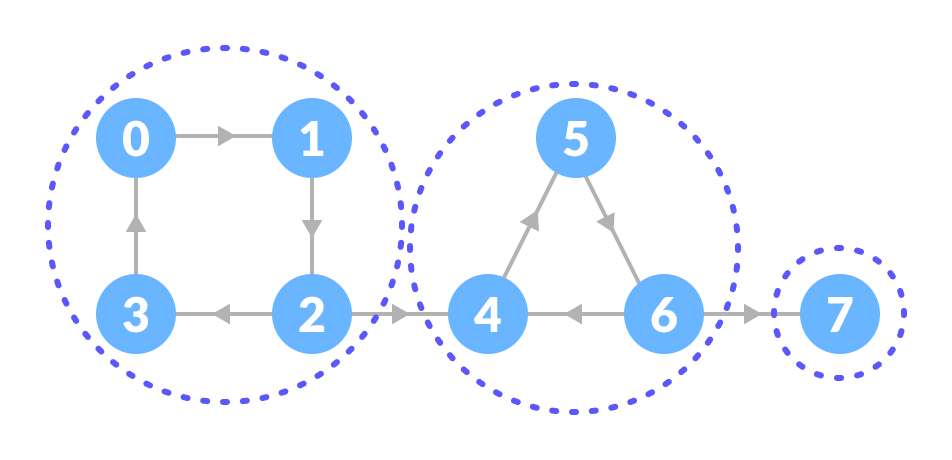You can observe that in the first strongly connected component, every vertex can reach the other vertex through the directed path.

These components can be found using Kosaraju's Algorithm.

## Kosaraju's Algorithm

Kosaraju's Algorithm is based on the depth first search algorithm implemented twice.

Three steps are involved.

1. Perform a depth first search on the whole graph.

Let us start from vertex-0, visit all of its child vertices and mark the visited vertices as done. If a vertex leads to an already visited vertex, then push this vertex to the stack.

For example: Starting from vertex-0, go to vertex-1, vertex-2 and then to vertex-3. Vertex-3 leads to already visited vertex-0, so push the source vertex (ie. vertex-3) into the stack.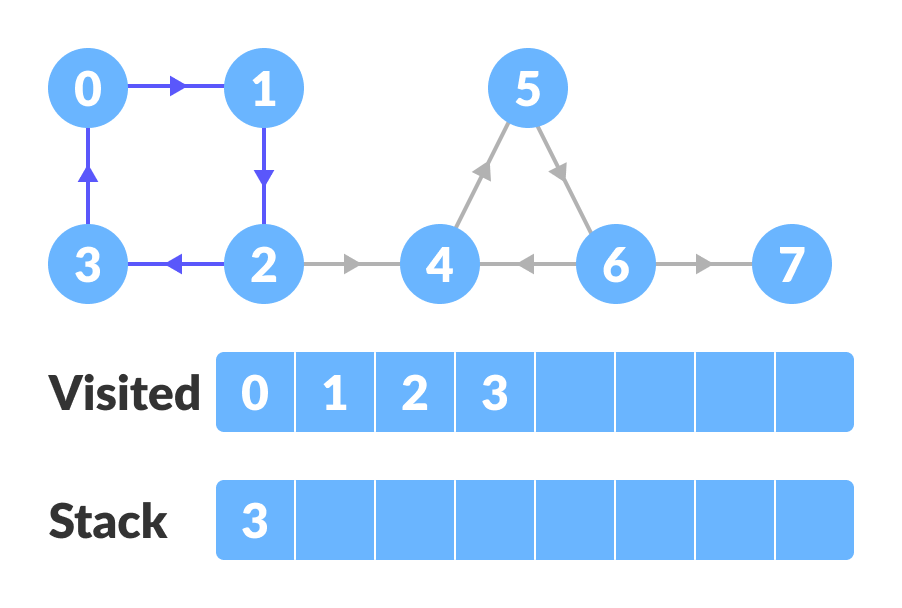Go to the previous vertex (vertex-2) and visit its child vertices i.e. vertex-4, vertex-5, vertex-6 and vertex-7 sequentially. Since there is nowhere to go from vertex-7, push it into the stack.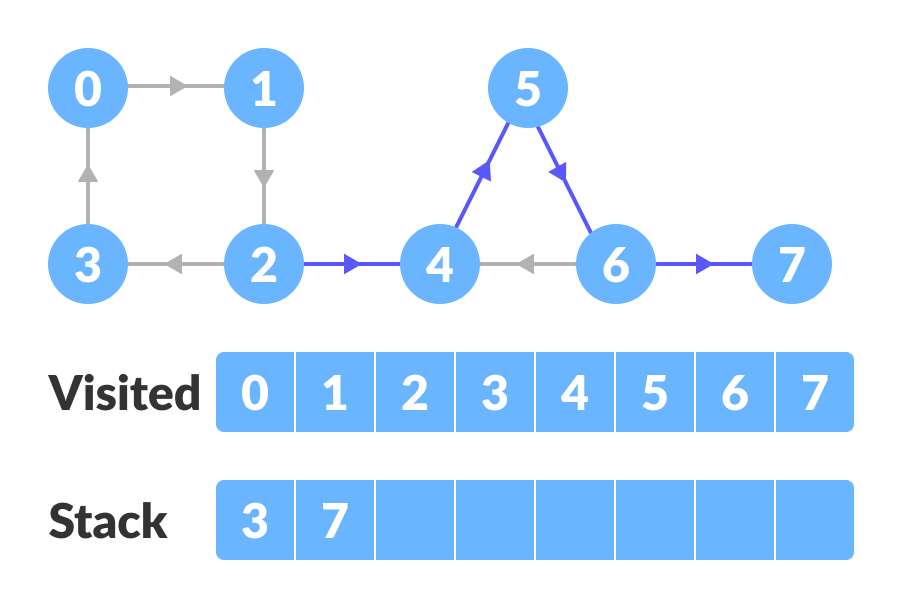Go to the previous vertex (vertex-6) and visit its child vertices. But, all of its child vertices are visited so, push it into the stack.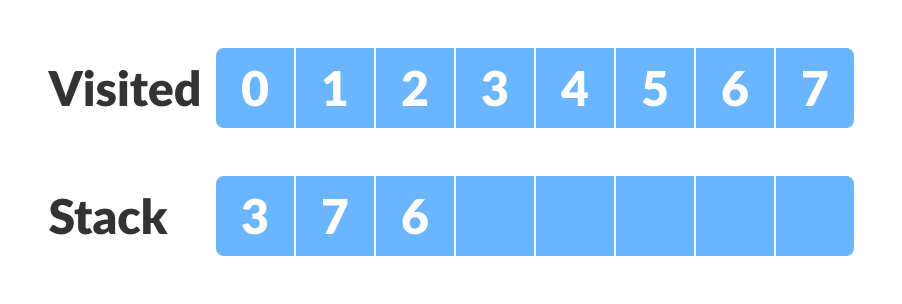Similarly, a final stack is created.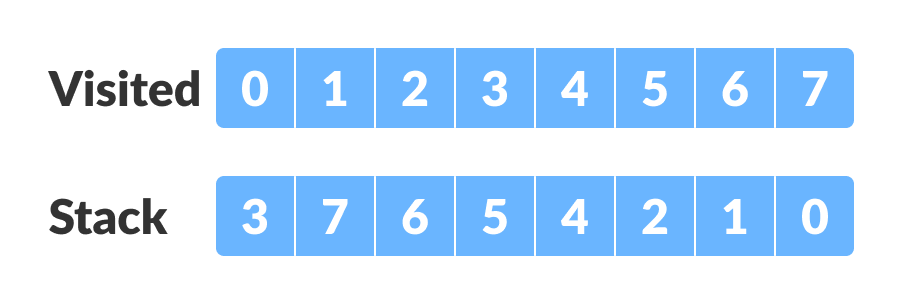2. Reverse the original graph.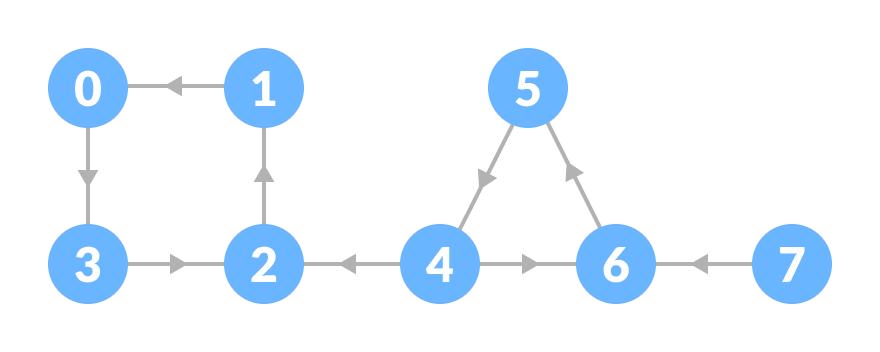3. Perform depth first search on the reversed graph.

Start from the top vertex of the stack. Traverse through all of its child vertices. Once the already visited vertex is reached, one strongly connected component is formed.

For example: Pop vertex-0 from the stack. Starting from vertex-0, traverse through its child vertices (vertex-0, vertex-1, vertex-2, vertex-3 in sequence) and mark them as visited. The child of vertex-3 is already visited so, these visited vertices form one strongly connected component.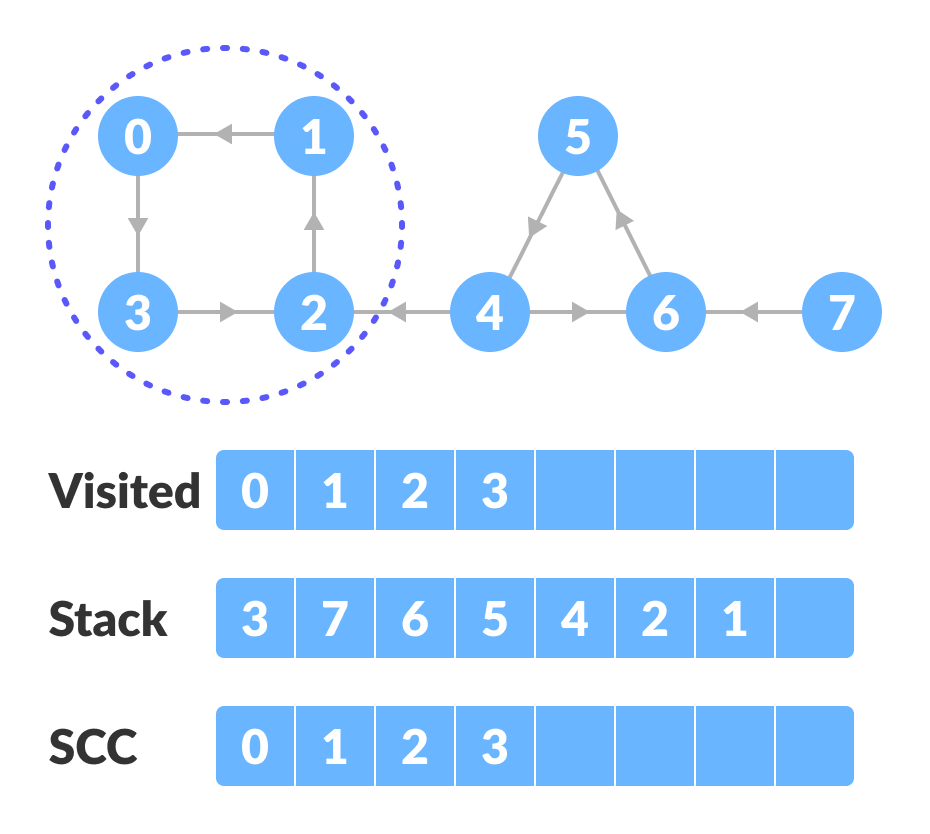Go to the stack and pop the top vertex if already visited. Otherwise, choose the top vertex from the stack and traverse through its child vertices as presented above.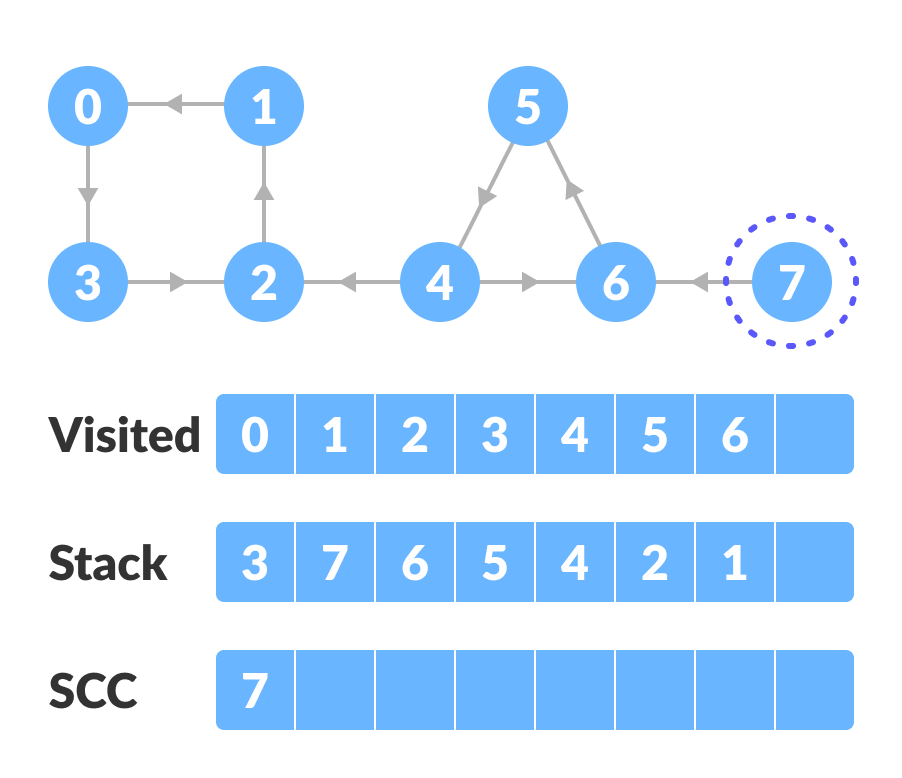4. Thus, the strongly connected components are: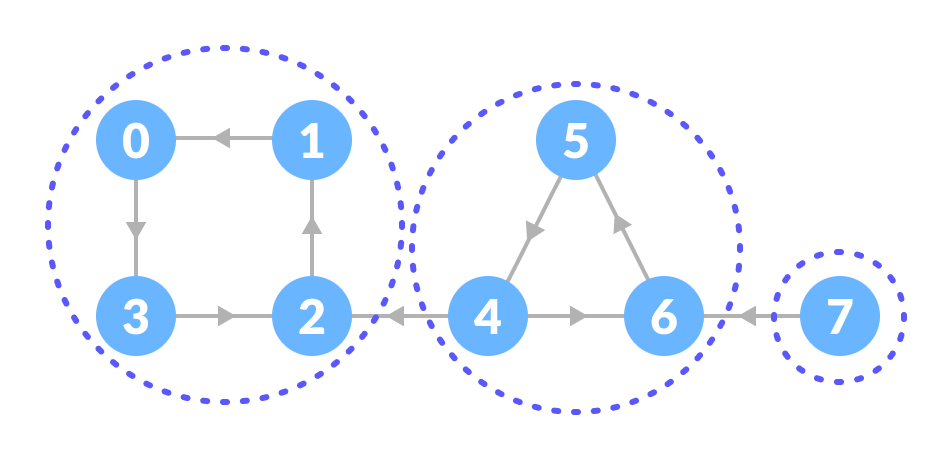## Python, Java, C++ examples

``````# Kosaraju's algorithm to find strongly connected components in Python

from collections import defaultdict

class Graph:

def __init__(self, vertices):
self.V = vertices
self.graph = defaultdict(list)

self.graph[u].append(v)

def DFS(self, v, visited):
visited[v] = True
print(v, end='')
for i in self.graph[v]:
if not visited[i]:
self.DFS(i, visited)

def fillOrder(self, v, visited, stack):
visited[v] = True
for i in self.graph[v]:
if not visited[i]:
self.fillOrder(i, visited, stack)
stack = stack.append(v)

def Transpose(self):
g = Graph(self.V)

for i in self.graph:
for j in self.graph[i]:
return g

def printSCC(self):
stack = []
visited = [False] * (self.V)

for i in range(self.V):
if not visited[i]:
self.fillOrder(i, visited, stack)

gr = self.Transpose()

visited = [False] * (self.V)

while stack:
i = stack.pop()
if not visited[i]:
gr.DFS(i, visited)
print("")

g = Graph(8)

print("Strongly Connected Components:")
g.printSCC()``````
``````// Kosaraju's algorithm to find strongly connected components in Java

import java.util.*;

class Graph
{
private int V;

Graph(int v)
{
V = v;
for (int i=0; i<v; ++i)
}

void DFSUtil(int v,boolean visitedVertices[])
{
visitedVertices[v] = true;
System.out.print(v + " ");
int n;

while (i.hasNext())
{
n = i.next();
if (!visitedVertices[n])
DFSUtil(n,visitedVertices);
}
}

Graph Transpose()
{
Graph g = new Graph(V);
for (int v = 0; v < V; v++)
{
while(i.hasNext())
}
return g;
}

void fillOrder(int v, boolean visitedVertices[], Stack stack)
{
visitedVertices[v] = true;

while (i.hasNext())
{
int n = i.next();
if(!visitedVertices[n])
fillOrder(n, visitedVertices, stack);
}
stack.push(new Integer(v));
}

void printSCC()
{
Stack stack = new Stack();

boolean visitedVertices[] = new boolean[V];
for(int i = 0; i < V; i++)
visitedVertices[i] = false;

for (int i = 0; i < V; i++)
if (visitedVertices[i] == false)
fillOrder(i, visitedVertices, stack);

Graph gr = Transpose();

for (int i = 0; i < V; i++)
visitedVertices[i] = false;

while (stack.empty() == false)
{
int v = (int)stack.pop();

if (visitedVertices[v] == false)
{
gr.DFSUtil(v, visitedVertices);
System.out.println();
}
}
}

public static void main(String args[])
{
Graph g = new Graph(8);

System.out.println("Strongly Connected Components:");
g.printSCC();
}
}``````
``````// Kosaraju's algorithm to find strongly connected components in C++

#include <iostream>
#include <list>
#include <stack>

using namespace std;

class Graph
{
int V;
void fillOrder(int v, bool visitedVertices[], stack<int> &Stack);
void DFS(int v, bool visitedVertices[]);
public:
Graph(int V);
void printSCC();
Graph transpose();
};

Graph::Graph(int V)
{
this->V = V;
}

void Graph::DFS(int v, bool visitedVertices[])
{
visitedVertices[v] = true;
cout << v << " ";

list<int>::iterator i;
if (!visitedVertices[*i])
DFS(*i, visitedVertices);
}

Graph Graph::transpose()
{
Graph g(V);
for (int v = 0; v < V; v++)
{
list<int>::iterator i;
{
}
}
return g;
}

{
}

void Graph::fillOrder(int v, bool visitedVertices[], stack<int> &Stack)
{
visitedVertices[v] = true;

list<int>::iterator i;
if(!visitedVertices[*i])
fillOrder(*i, visitedVertices, Stack);

Stack.push(v);

}

void Graph::printSCC()
{
stack<int> Stack;

bool *visitedVertices = new bool[V];
for(int i = 0; i < V; i++)
visitedVertices[i] = false;

for(int i = 0; i < V; i++)
if(visitedVertices[i] == false)
fillOrder(i, visitedVertices, Stack);

Graph gr = transpose();

for(int i = 0; i < V; i++)
visitedVertices[i] = false;

while (Stack.empty() == false)
{
int v = Stack.top();
Stack.pop();

if (visitedVertices[v] == false)
{
gr.DFS(v, visitedVertices);
cout << endl;
}
}
}

int main()
{
Graph g(8);

cout << "Strongly Connected Components:\n";
g.printSCC();
}``````

## Kosaraju's Algorithm Complexity

Kosaraju's algorithm runs in linear time i.e. `O(V+E)`.

## Strongly Connected Components Applications

• Vehicle routing applications
• Maps
• model checking in formal verification